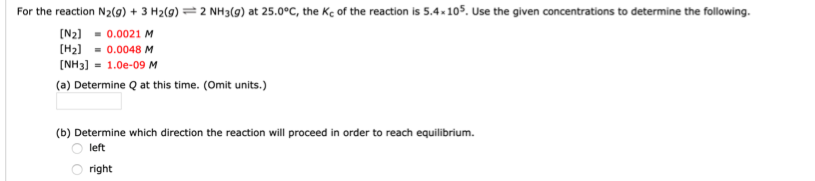# For the reaction N2(g)+ 3 H2(g) - 2 NH3(g) at 25.0°C, the Kc of the reaction is 5.4.105. Use the given concentrations to determine the following. [N2]=0.0021 M [H2]=0.0048 M [NH3]=1.0e-09 M (a) Determine Q at this time. (Omit units.) (b) Determine which direction the reaction will proceed in order to reach equilibrium. left right